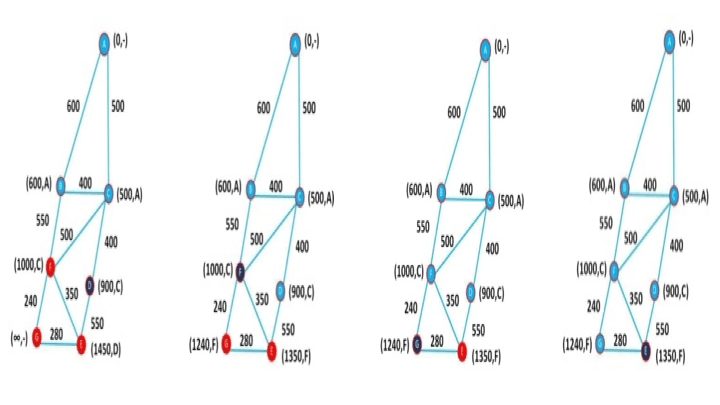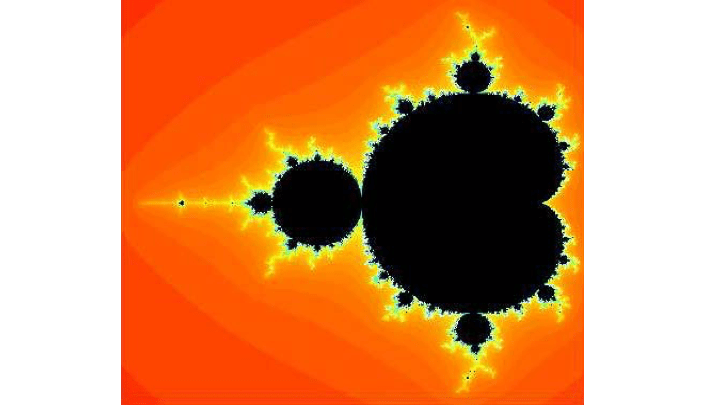# A week project on Mathematics for high school graduatesThe Laboratory for Mathematical Engineering (LEMA) of the School of Engineering of the Polytechnic Institute of Porto is committed to attract new students to mathematical areas. With this in mind, we promote several one-week projects on Applied Mathematics for high school graduates. These students are the next year candidates for a college degree and our goal is to try to influence them on deciding for a degree on mathematics or similar.

Projects focus on simple real world applications, with an effort on showing students the importance of different fields of Mathematics in our daily life. Existing since 2009, they give students the opportunity to be integrated on a research group and provide them a hands-on experience on research areas that are usually introduced in higher education levels. Projects cover different topics such as fractals, cryptography, neural networks, Bayesian statistics, graph theory, or optimisation. Here are some examples:

#### How can a GPS find the shortest path between two locations?

Students are introduced to the basics of Graph Theory and algorithms to find the shortest path between two points, under certain conditions. They use the theoretical concepts to build a simple program to solve the proposed problem.Figure 1. Partial illustration of Dijkstra’s algorithm.

#### Coding messages using concepts of linear algebra.

In this project, matrices and their properties are used to code and decode written messages. Students produce a simple program that, given a message, uses a matrix to code it and the inverse to decode it.

#### What is the size of Portugal?

Students discuss the hypothesis that the length of the border between Portugal and Spain does not exist. They are introduced to the notion of fractal structures and with the fact that many natural borders have the properties of these structuresFigure 2. Example of a fractal produced by a student.

#### Time traveling with 3D curves.

In this project, students are introduced with a collection of XIX century artefacts, belonging to the ISEP Museum. All these objects represent ruled surfaces that can be described as moving straight lines. The objective is to use descriptive geometry formulas to describe these surfaces and to simulate them with a graphical software.Figure 3. One of the museum’s artefacts.

#### Who is the crime suspect?

When a crime is committed, several evidences are collected. Forensic scientists use these evidences to statistically evaluate the probability of a suspect being responsible for the act. The same problem occurs with paternity identification. Students are introduced to Bayesian Theory and how they can use it to solve such kind of problems.

#### Mathematics and the intelligence of neural networks.

With an introduction to neural networks and a hands-on software implementation of a simple classification example using a very basic neural network, students are introduced to this field of research and are able to understand the importance of mathematics on how networks learn from examples and extrapolate to future observations.Figure 4. The result of a simple 2-class classification problem.

#### Conclusion

The outcome of these yearly events has been much rewarding. Students that apply for this kind of projects have an appetence for mathematics and are, therefore, highly motivated for subjects related with this area of research. The number of candidates is always much higher than the number of available places showing that these initiatives are specially appreciated by students that come from different regions to an intensive one-week project that, helpfully, would improve their insight on mathematical applications.

Jorge Santos (LEMA/ISEP/IPP)

LEMA – Laboratory for Mathematical Engineering
Rua Dr. António Bernardino de Almeida, 431
4249-015 Porto – PORTUGAL
tel. +351 228 340 500 | fax +351 228 321 159